﻿ 音频感知哈希闭环检测的无人机仿生声呐SLAM算法研究
«上一篇文章快速检索 高级检索

 智能系统学报2019, Vol. 14Issue (2): 338-345  DOI: 10.11992/tis.2017080180

### 引用本文HU Wenchao, SUN Xinzhu, CHEN Mengyuan. Research on BATSLAM algorithm for UAV based on audio perceptual hash closed-loop detection[J]. CAAI Transactions on Intelligent Systems, 2019, 14(2): 338-345. DOI: 10.11992/tis.201708018.### 文章历史

Research on BATSLAM algorithm for UAV based on audio perceptual hash closed-loop detection
HU Wenchao , SUN Xinzhu, CHEN Mengyuan
Anhui Key Laboratory of Electric Drive and Control, Anhui Polytechnic University, Wuhu 241000, China
Abstract: Aiming at the problem of constructing two-dimensional empirical graph based on SLAM technology at certain heights ,on the basis of RatSLAM, using the bionic sonar system to replace the visual sensor's BatSLAM mode and audio perceptual hash closed-loop detection, realizing two-dimentional empirical graph optimization under dark conditions. The BatSLAM model uses Sum of Absolute Difference (SAD) image processing methods to update the bionic sonar template. This method only judges whether the appearance of the two cochlear images is consistent, and does not have geometric processing and feature extraction. Because the cochlear images produce various noises during the acquisition and transmission, There are some differences in cochlear maps obtained at the same position. which can lead to the distortion of the constructed empirical map. This article is based on BatSLAM, The audio perceptual hash algorithm is used to extract features of cochlea ,and according to closed-loop detection. The improved algorithm not only considers the appearance, but also considers the energy difference between adjacent bands. By improving the accuracy of closed-loop detection, to improve the experience map of the distortion problem. Simulation experiment shows,the BatSLAM model based on audio perceptual hash closed-loop detection is adopted, it not only realizes the construction of two-dimensional experience map under certain height and dark conditions of UAV, but also improves the accuracy of the closed-loop detection, improving the experience map of the distortion problem and implementing the optimization of experience graph.
Key words: simultaneous localization and mapping    audio perceptual hash    unmanned aerial vehicle    closed-loop detection    Experience diagram    bionic sonar    sum of absolute difference    Cochlear map

1 算法原理与设计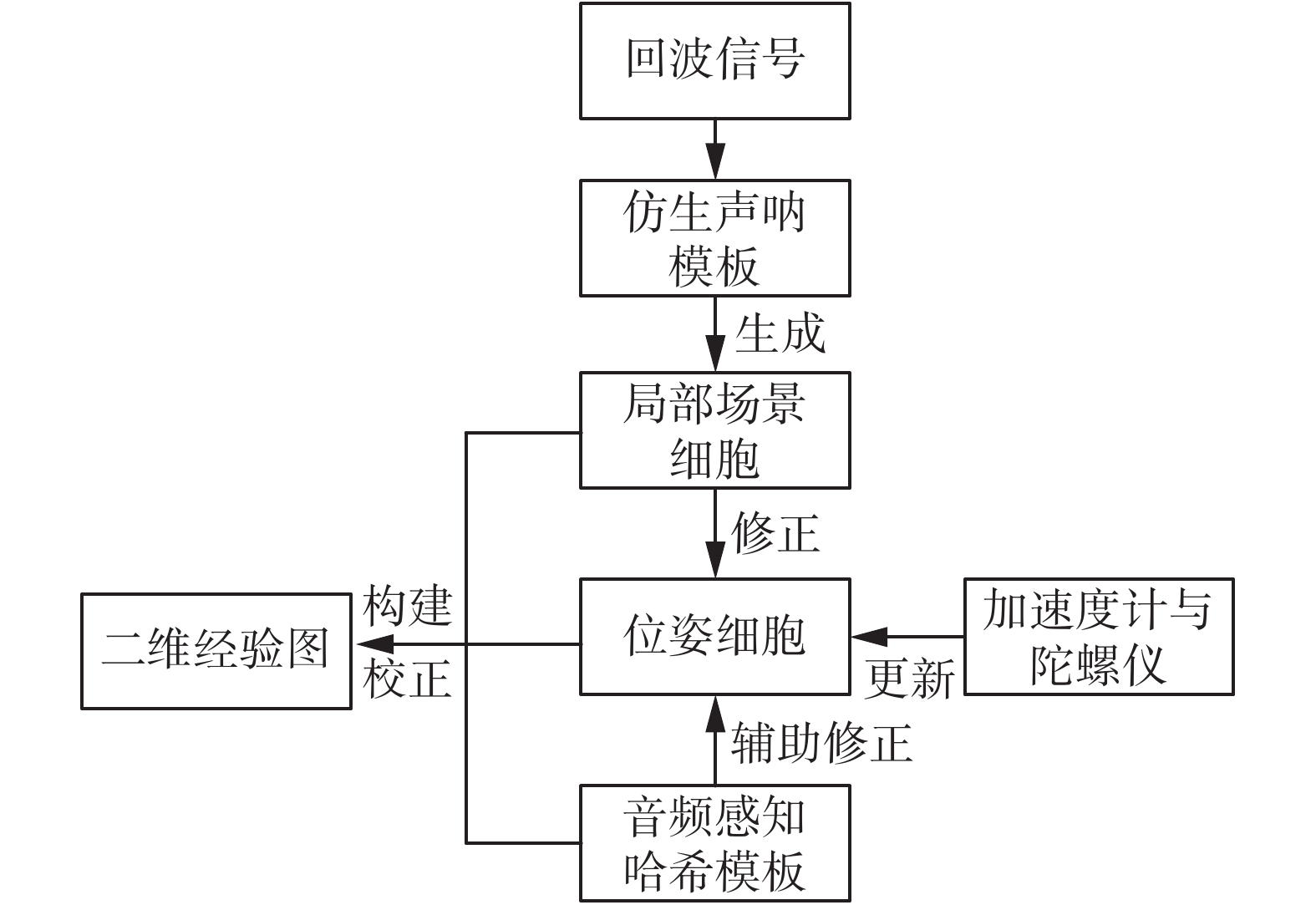Download: 图 1 改进后的BatSLAM算法的结构框图 Fig. 1 Structure block diagram of the improved BatSLAM algorithm
1.1 仿生声呐模型

 $H_{\rm{ERTF}}^L(f,\theta ) = {H_e}(f,\theta ) \cdot H_R^L(f,\theta )$ (1)
 $H_{\rm{ERTF}}^R(f,\theta ) = {H_e}(f,\theta ) \cdot H_R^R(f,\theta )$ (2)

 $x ^L(t) = \sum\limits_{i = 1}^{ne} {h_e^L} (t;{\theta _i}) * {s }(t - {\delta _i})$ (3)
 $x ^R(t) = \sum\limits_{i = 1}^{ne} {h_e^R} (t;{\theta _i}) * {s }(t - {\delta _i})$ (4)

 ${h^{gt}}(t;{f_c}(n)) = {t^3} \cdot {{\rm{e}}^{ - 2{\text{π}} b \cdot t}}\cos (2{\text{π}} {f_c}(n) \cdot t)$ (5)

 $X_L^{}(t) = x_L(t) * {h^{gt}}(t;{f_c}(n)) * {u^{lp}}(t)$ (6)
 $X_R^{}(t) = x_R(t) * {h^{gt}}(t;{f_c}(n)) * {u^{lp}}(t))$ (7)

 ${{X}} ^{gt,SS} = \left[ {X_L^{gt} * {G^S} \quad X_R^{gt} * {G^S}} \right]$ (8)

 ${\overline X } ^{gt,SS} = \frac{{{{X}} ^{gt,SS}}}{{\sqrt {\sum\nolimits_t {\sum\nolimits_f {{{\left. {\left| {{{X}} ^{gt,SS}} \right.} \right|}^2}} } } }}$ (9)

 ${G_{cur}} = {\overline X } ^{gt,SS}$ (10)
1.2 位姿感知细胞

1) CAN模型

 ${\varepsilon _{a,b,c}} = {{\rm{e}}^{ - ({a^2} + {b^2})/{k_m}}} \cdot {{\rm{e}}^{ - ({c^2})/{k_n}}}$ (11)

 $\Delta {P_{{x'}{y'}{\theta '}}} = \sum\limits_{a = 0}^{{N_{{x'}}}} {\sum\limits_{b = 0}^{{N_{{y'}}}} {\sum\limits_{c = 0}^{{N_{{\theta '}}}} {{\varepsilon _{(a - {x'})(b - {y'})(c - {\theta '})}}} } } {P_{abc}}$ (12)

 $P_{{x'y'\theta '}}^{t + 1} = \max [P_{{x'y'\theta '}}^t + \varphi (P_{{x'y'\theta '}}^t - \max (P)),0]$ (13)

2)直观的路径积分

 $P_{_{x'y'\theta '}}^{t + 1} = \sum\limits_{a = \delta {x'}_0}^{\delta {x'}_0 + 1} {\sum\limits_{b = \delta {y'}_0}^{\delta {y'}_0 + 1} {\sum\limits_{c = \delta {\theta '}_0}^{\delta {\theta '}_0 + 1} {{P^t}({x'} + a)} } } ({y'} + b)({\theta '} + c)$ (14)

 $\left[ {\begin{array}{*{20}{c}} {\delta x_0'} \\ {\delta y_0'} \\ {\delta \theta _0'} \end{array}} \right] = \left[ {\begin{array}{*{20}{c}} {{k_{{x'}}}v\cos \theta } \\ {{k_{{y'}}}v\sin \theta } \\ {{k_{{\theta '}}}w} \end{array}} \right]$ (15)

3)仿生声呐模板修正位姿感知细胞

 $\beta _{i{x'}{y'}{\theta '}}^{t + 1} = \max ({\beta ^t}_{{x'}{y'}{\theta '}},\lambda {G_i}{P_{{x'}{y'}{\theta '}}})$ (16)

 ${d_i} = \sqrt {\sum {({U_{\rm{cur}}} - {U_i})} }$ (17)

 $\Delta {P_{{x'}{y'}{\theta '}}} = \sum\limits_i {{\beta ^{}}_{{x'}{y'}{\theta '}}} {G_i}$ (18)

 $\tau = {\alpha _t}\sqrt {\frac{{\sum\limits_{i = 1}^{{n_G}} {d_i^2} }}{{{n_G}}}}$ (19)

1.3 音频感知哈希算法修正位姿细胞

1)音频感知哈希

 ${\rm{ph}}={\rm{PH}}(n),\,\, n \in N$ (20)

2)音频感知哈希的生成

 $\begin{gathered} {W_{(n,L)}} = \sigma \sum\limits_{i = 1}^{80} {\sqrt {{{(S_n^{(r + m)})}^2}} } \\ r = (L - 1) \times 40;\,\, n = 1,2,\cdots ,8 \end{gathered}$ (21)

 $\begin{gathered} {R_k} = {\Delta ^2}{Q_k} = Q(i) - 2Q(i + 1) + Q(i + 2) \hfill \\ i = 1,2,\cdots ,6 \hfill \\ \end{gathered}$ (22)

 $Y(t,k) = \left\{ \begin{array}{l} 0,\,\,\,\,{R_k}(t) > 0\\ 1,\,\,\,\,{R_k}(t) \leqslant 0 \end{array} \right. \\$ (23)

$t = 1,2, \cdots ,6;k = 1,2, \cdots ,L/8$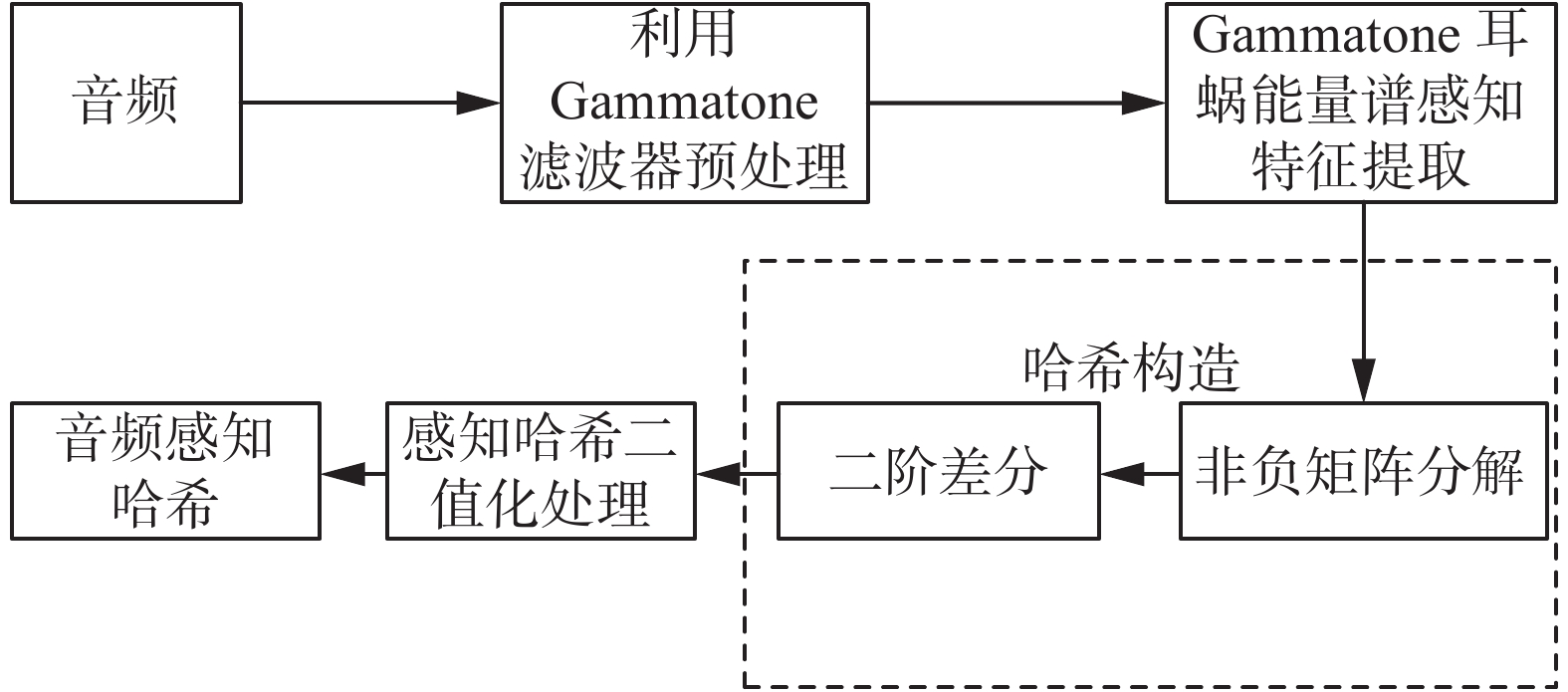Download: 图 2 音频感知哈希生成 Fig. 2 Audio perception Hashi generation

3)音频感知哈希模板修正

 $\beta _{i{x'}{y'}{\theta '}}^{t + 1} = \max ({\beta ^t}_{{x'}{y'}{\theta '}},\lambda {Y_i}{P_{{x'}{y'}{\theta '}}})$ (24)

 $\Delta {P_{{x'}{y'}{\theta '}}} = \sum\limits_i {{\beta ^{}}_{{x'}{y'}{\theta '}}} {Y_i}$ (25)

1.4 经历图的构建与校正

 ${e_{{x_i},{y_i},{\theta _i}}} = \langle {E_i},{P_{{x_i},{y_i},{\theta _i}}},{Y_i},{G_i}\rangle$ (26)

 ${E_i} = \left\{ {\begin{array}{*{20}{l}} {0}, \,\,\,\,\,\,\,\,{\text{其他}}\\ {\rm{2 - }}\displaystyle\frac{{\sqrt {{{(x_{pc}' - x_i')}^2} + {{(y_{pc}' - y_i')}^2}} }}{{{r_a}}}{\rm{ - }}\frac{{\left| {\theta _{pc}' - \theta _i'} \right|}}{{{\theta _a}}},\\ &\!\!\!\!\!\!\!\!\!\!\!\!\!\!\!\!\!\!\!\!\!\!\!\!\!\!\!\!\!\!\!\!\!{{\rm{G}}_{\rm{cur}}} = {G_i}{\text{或}}{Y_{\rm{cur}}} = {Y_i} \end{array}} \right.$ (27)

 $\Delta {p^i} = \alpha (\sum\limits_{j = 1}^{{N_f}} {({p^j} - {p^i} - \Delta {p^{ij}}) + } \sum\limits_{k = 1}^{{N_t}} {{p^k} - {p^i} - } \Delta {p^{ki}})$ (28)

2 实验与分析 2.1 硬件平台数据处理

 ${\rm{Alt}} = 44\;330 \cdot \left( {1 - {{\left( {\frac{P}{{{P_0}}}} \right)}^{\frac{1}{{5.255}}}}} \right)$

2.2 音频感知哈希构建基础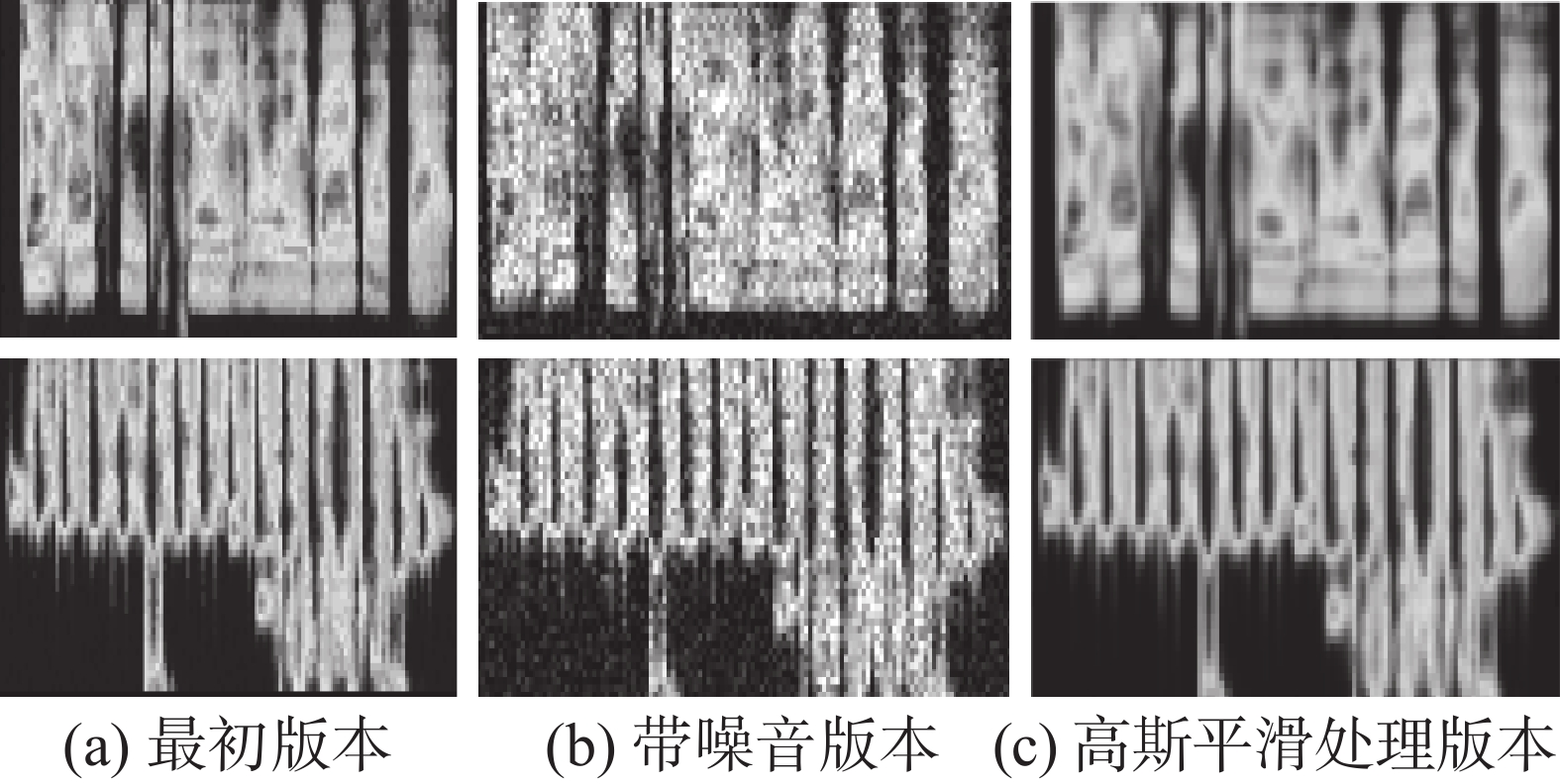Download: 图 3 最初音频和处理后的音频耳蜗能量谱比较 Fig. 3 Comparison of the energy spectrum between the initial and the processed audio cochlea.
2.3 不同噪音条件下改进算法与原算法的准确率−召回率对比试验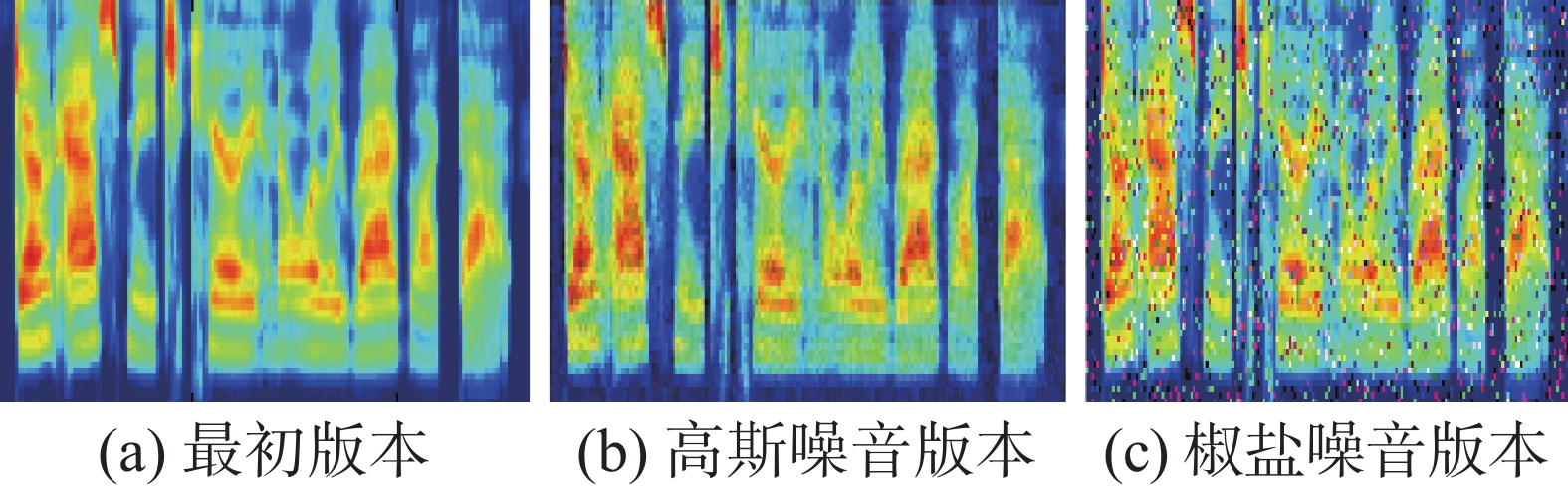Download: 图 4 不同噪音版本与原始版本耳蜗图对比 Fig. 4 Comparison of different noise versions and original versions of cochlea

 $p = {{\rm{TP}}/({{\rm{TP}} + {\rm{FP}}}})$ (30)
 $R = {{\rm{TP}}/( {{\rm{TP}} + {\rm{FN}}}})$ (31)表 1 BatSLAM在不同条件下的准确率、召回率 Tab.1 The accuracy and recall rate of BatSLAM under different conditions表 2 基于音频感知哈希的BatSLAM在不同条件下的准确率、召回率 Tab.2 The accuracy and recall rate of BatSLAM based on audio perception Hashi under different conditions

2.4 改进算法与原算法经历图对比实验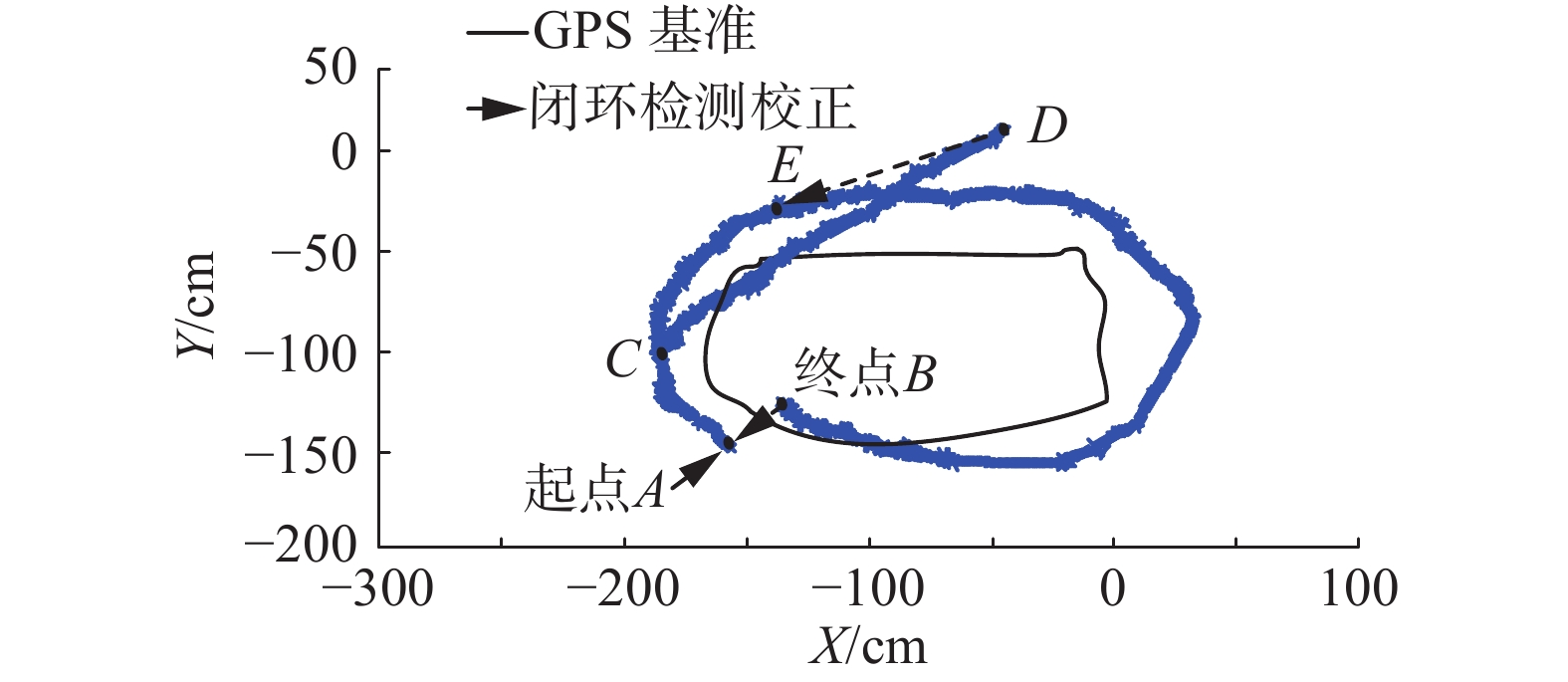Download: 图 5 BatSLAM经历图 Fig. 5 BatSLAM experience diagram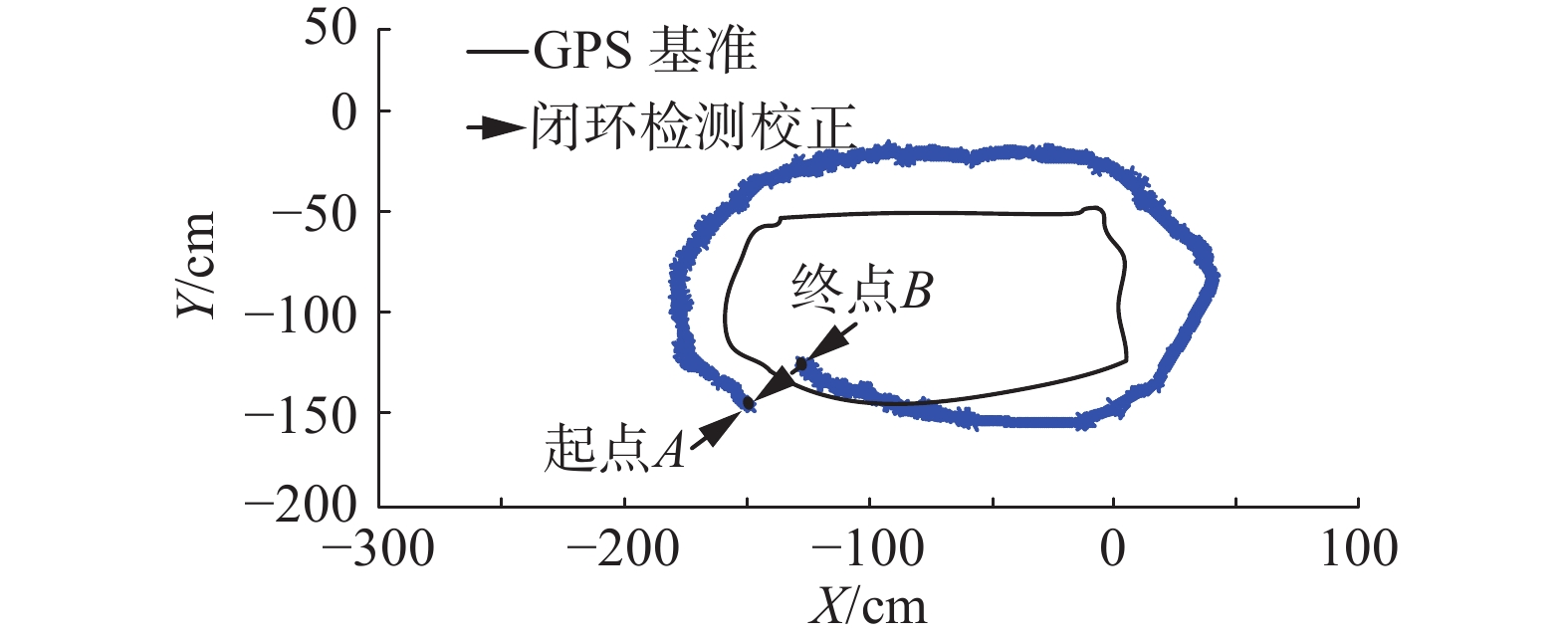Download: 图 6 基于音频感知哈希闭环检测的BatSLAM经历图 Fig. 6 BatSLAM experience graph based on audio perception Hashi closed loop detection
3 结束语

  MUR-ARTAL R, MONTIEL J M M, TARDóS J D. ORB-SLAM: a versatile and accurate monocular SLAM system[J]. IEEE transactions on robotics, 2015, 31(5): 1147-1163. DOI:10.1109/TRO.2015.2463671 (0)  HUANG G P, MOURIKIS A I, ROUMELIOTIS S I. A quadratic-complexity observability-constrained unscented Kalman filter for SLAM[J]. IEEE transactions on robotics, 2013, 29(5): 1226-1243. DOI:10.1109/TRO.2013.2267991 (0)  张凤, 黄陆君, 袁帅, 等. NLOS环境下基于EKF的移动机器人定位研究[J]. 控制工程, 2015, 22(1): 14-19. ZHANG Feng, HUANG Lujun, YUAN Shuai, et al. Reach on robot localization based on EKF in NLOS environment[J]. Control engineering of China, 2015, 22(1): 14-19. (0)  刘卫华, 王希彬, 周强. 基于容积粒子滤波的无人机FastSLAM算法研究[J]. 系统仿真技术, 2014, 10(3): 234-238. LIU Weihua, WANG Xibin, ZHOU Qiang. FastSLAM algorithm based on cubature particle filtering algorithm for UAV[J]. System simulation technology, 2014, 10(3): 234-238. DOI:10.3969/j.issn.1673-1964.2014.03.010 (0)  丛楚滢, 王从庆, 丁臻极, 等. 一种小型无人机的FastSLAM算法[J]. 华中科技大学学报(自然科学版), 2015, 43(S1): 420-423. CONG Chuying, WANG Congqing, DING Zhenji, et al. A FastSLAM algorithm for small unmanned aerial vehicle[J]. Journal of Huazhong university of science and technology (nature science edition), 2015, 43(S1): 420-423. (0)  吕品, 赖际舟, 杨天雨, 等. 基于气动模型辅助的四旋翼飞行器室内自主导航方法[J]. 航空学报, 2015, 36(4): 1275-1284. LYU Pin, LAI Jizhou, YANG Tianyu, et al. Autonomous navigation method aided by aerodynamics model for an indoor quadrotor[J]. Acta aeronautica et astronautica sinica, 2015, 36(4): 1275-1284. (0)  蒙山, 唐文名. 单目SLAM直线匹配增强平面发现方法[J]. 北京航空航天大学学报, 2017, 43(4): 660-666. MENG Shan, TANG Wenming. Monocular SLAM plane discovery method enhanced by line segments matching[J]. Journal of Beijing university of aeronautics and astronsutics, 2017, 43(4): 660-666. (0)  余小欢, 韩波, 张宇, 等. 基于双目视觉的微型无人机室内三维地图构建[J]. 信息与控制, 2014, 43(4): 392-397. YU Xiaohuan, HAN Bo, ZHANG Yu, et al. Binocular stereo vision based 3D mapping for micro aerial vehicles in an indoor environment[J]. Information and control, 2014, 43(4): 392-397. (0)  吕科, 施泽南, 李一鹏. 微型无人机视觉定位与环境建模研究[J]. 电子科技大学学报, 2017, 46(3): 543-548. Lü Ke, SHI Zenan, LI Yipeng. Visual localization and environment mapping for micro aerial vehicles[J]. Journal of university of electronic science and technology of China, 2017, 46(3): 543-548. DOI:10.3969/j.issn.1001-0548.2017.03.011 (0)  王国胜, 郭峰, 刘峰. 无人飞行器视觉SLAM特征检测和匹配算法研究[J]. 计算机测量与控制, 2015, 23(7): 2453-2455. WANG Guosheng, GUO Feng, LIU Feng. Research on feature detection and matching algorithm of unmanned aerial vehicles visual SLAM[J]. Computer measurement & control, 2015, 23(7): 2453-2455. (0)  王闯, 卢健, 黄杰. AUV同时定位与跟踪算法研究[J]. 西安工程大学学报, 2014, 28(5): 556-562. WANG Chuang, LU Jian, HUANG Jie. Algorithms research of simultaneous localization and tracking for AUVs[J]. Journal of Xi’an polytechnic university, 2014, 28(5): 556-562. DOI:10.3969/j.issn.1674-649X.2014.05.008 (0)  STECKEL J, PEREMANS H. Spatial sampling strategy for a 3D sonar sensor supporting BatSLAM[C]//Proceedings of 2015 IEEE/RSJ International Conference on Intelligent Robots and Systems. Hamburg, Germany, 2015: 723–728. (0)  TUBMAN R, POTGIETER J, ARIF K M. Efficient robotic SLAM by fusion of RatSLAM and RGBD-SLAM[C]//Proceedings of the 201623rd International Conference on Mechatronics and Machine Vision in Practice. Nanjing, China, 2016: 1–6. (0)  STECKEL J, PEREMANS H. BatSLAM: simultaneous localization and mapping using biomimetic sonar[J]. PLoS One, 2013, 8(1): e54076. DOI:10.1371/journal.pone.0054076 (0)  YU Hongshan, ZHANG Yuan, WANG Yaonan, et al. Fast and robust frontier line segment extracting method based on FCM for robot exploration[C]//Proceedings of 2013 Chinese Automation Congress. Changsha, China, 2013: 685–690. (0)  王伟, 陈志高, 孟宪凯, 等. 基于熵的音频指纹检索技术研究与实现[J]. 计算机科学, 2017, 44(S1): 551-556. WANG Wei, CHEN Zhigao, MENG Xiankai, et al. Research and implementation of identifying music through performances using entropy based audio-fingerprint[J]. Computer science, 2017, 44(S1): 551-556. DOI:10.11896/j.issn.1002-137X.2017.6A.123 (0)